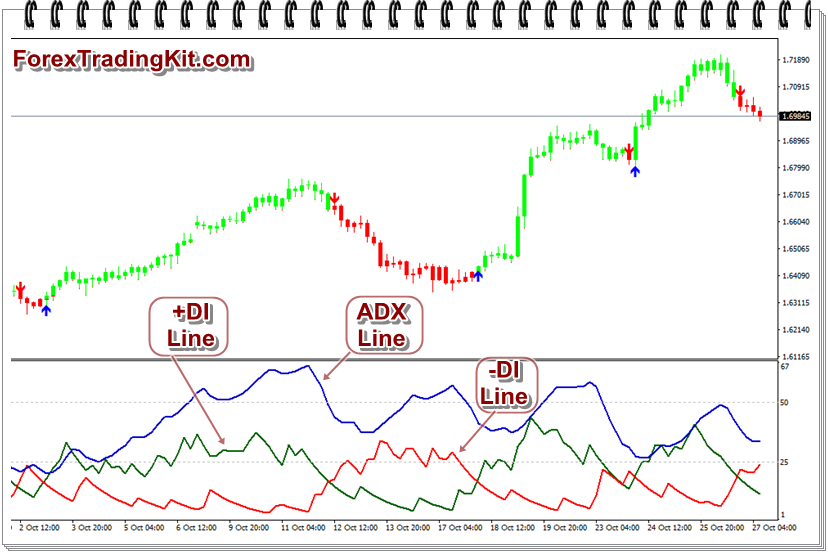## Thursday, May 10, 2018

### Guide to ADX Indicator (Average Directional Index)

J. Welles Wilder developed the Average Directional Index ( ADX) indicator to determine the strength of the trend, whether the trend will develop further or gradually decline. The ADX indicator allows analyzing market trends and making trading decisions, including in the forex market.

Calculate positive and negative directional movement (Directional Movement or DM) - + DMj and -DMj

If Highj (maximum current bar)> Highj-1 (maximum of the previous bar),
then + DMj = Highj - Highj-1, or + DMj = 0
If Lowj (at least the current bar) <Lowj-1 (low of the previous bar),
then -DMj = Lowj-1-Lowj, otherwise -DMj = 0
if + DMj> -DMj,
then -DMj = 0
if -DMj> + DMj,
then + DMj = 0
if + DMj = -DMj,
then + DMj = 0 , -DMj = 0

Determines the true range - TRj (True Range)
TR = the maximum of the modules of the three values ??of
| High - Low |, | High - Close j-1 |, | Low - Close j-1 |.
Where Close j-1 is the closing price of the previous period.

Note: at forex in the absence of price breaks the maximum in most cases will be | High - Low |.

The positive direction indicator and the negative direction indicator - + DIj and -DIj (Directional Index) are determined .

+ DIj = Exponential Moving Averagej (+ SDI, N)
-DIj = Exponential Moving Averagej (-SDI, N)

where, if TRj is not = 0,
then + SDIj = + DMj / TRj;
-SDIj = -DMj / TRj
if TRj = 0,
then + SDIj = 0,
-SDIj = 0,

The mean directional motion is determined - ADXj.
ADXj = Exponential Moving Average (DX, N)
Where DXj is calculated by the formula

The ADX itself looks like this.ADX Indicator

Actually, the ADX indicator refers to the oscillator class , which ranges from 0 to 100. Although the movement of the ADX indicator is in the range from 0 to 100, the movement above the 60 mark is quite rare.

A value below 20 signals a weak trend, a value above 40 signals a strong trend. Data above 40 can show both a strong downtrend and a strong uptrend.

The ADX indicator can also be used to identify potential changes in the market. When the indicator reading crosses the limit of 20 from the bottom up - this can indicate a trend change and its further development. When the indicator shows a value less than 40, falling from a higher level, this may mean that the trend has lost power.

The ADX indicator is derived from the other two, which are also developed by Wilder. One indicator is called a Positive Directional Indicator (in most cases, sometimes referred to as "+ DI"), the second indicator is a Negative Directional Indicator (denoted as "-DI"). On the chosen time interval + DI- shows the strength of the movements up, and -DI shows the strength of the movements down for a certain period.

The ADX indicator shows the strength of the trend and combines + DI and -DI, then smoothing the data of the moving average. Since ADX uses the data + DI and -DI does not indicate the direction of the trend.

## How to use ADX indicator

• In its basic form, signals for buying and selling come at the intersections + DI / -DI. The buy signal comes when + DI crosses from below-DI, and the signal to the sale is intersection by the indicator -DI indicator + DI from top to bottom.

• For a trader, it will be important to catch the trend at an early stage of development and the ADX indicator should help it when achieving its goal and getting the benefits of making deals.

To catch the trend in the early stages, you need to look at currency pairs, where the ADX crosses 20 from the bottom up. Accordingly, the fall of ADX from below 40 signals that the current trend is weakened and the trading range may start. Thus, the ADX indicator itself shows the presence or absence of a trend, and other indicators can be used to determine the entry points and the direction of entry.

The signal to the input fed by the intersection of the indicators -DI and + DI is often false when the currency pair is in the trading range.